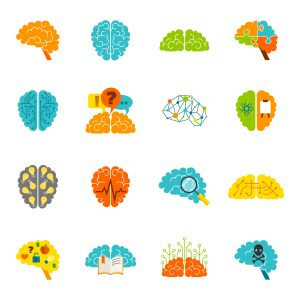## Learning Disabilities and Mathematics

Learning disabilities (LDs) are brain-based difficulties that reflect impairments in one or more of the psychological processes. It is estimated that between 3 and 9% of students have LDs in mathematics [i].

### The Processing Areas and Mathematics

LDs that impact mathematics learning are diverse, and may take many different forms. This section of the module describes how difficulties in the various psychological processes may lead to math challenges, and is based on information compiled from the York Waterfall Chart for Math.

Students with phonological processing difficulties may struggle with the following math-related tasks:

• Recognizing the relationship between the word (e.g., ten) and the symbol (e.g., 10)
• Decoding/reading math text (e.g., instructions, word walls, problems, inquiry questions, labels on diagrams and graphs)

Math is also affected by language processing difficulties, which may cause challenges in understanding:

• Mastering number facts and formulas for fluency
• Understanding mathematics conceptually and the use of math vocabulary (e.g., area, numerator, denominator, names of numbers, magnitude, negative, slope)
• Following instructions and understanding the steps of a problem solving approach when the steps are presented
• Comprehending word problems and extracting important ideas to begin solving
• Expressing mathematical thinking using multiple modalities (e.g., manipulatives, verbal explanation, visual representation)

For more information on phonological and language processing in the mathematics classroom, click here to access the webinar recording The SLP in the Math Class – Empowering Math Learners Through Collaboration between Educators and Speech Language Pathologists.

Challenges with visual-motor skills may lead to difficulties with:• Lining up columns
• Composing and drawing figures, shapes, and graphs
• Organizing and making good use of space on paper

Visual-spatial processing difficulties may impair mathematical abilities, because of challenges with:

• Understanding visual patterns (e.g., linear growing patterns) and picking out important visual details
• Understanding and recognizing the meaning of visuals presented (e.g., objects, diagrams, charts, graphs)
• Understanding number relationships and quantity (e.g., visualizing numbers, money, negative numbers)
• Understanding measurement relationships (e.g., distance, time, volume)
• Reasoning spatially (e.g., visualizing, scaling up and down, manipulating objects, mentally transforming objects)
• Accessing excessive text and/or visuals on a page
• Including and aligning math symbols (e.g., dollar signs, units, operations)

Students with memory challenges may experience difficulties:

• Remembering number facts and steps involved in computation
• Extracting information to be used in word problems
• Following multistep instructions
• Holding information in mind temporarily while simultaneously performing another problem solving task (e.g., regrouping, estimating, lining up math problems in appropriate columns)
• Recalling algorithms, formulas and/or a sequence of numbers with accuracy when shared orally

Processing speed may affect math because of difficulties with:

• Quickly, fluently and efficiently retrieving consolidated math facts
• Reading math questions or problems fluently
• Finishing work on time as output may be slow and laborious
• Completing sample problems during class time
• Performing basic arithmetic calculations in a timed format
• Recognizing simple visual patterns and scanning visual information quickly

Challenges with executive function may lead to difficulties with:

• Managing multistep problem solving
• Choosing the most appropriate strategies or operational processes (e.g., BEDMAS, balancing equations, linear regression)
• Revising their strategy or plan in the face of feedback, challenges, mistakes or new information
• Representing mathematical thinking so that it can be followed by themselves and others
• Managing mistakes (e.g., frequently erasing work)
• Showing or sharing how they solved a problem
• Knowing whether an answer is reasonable
• Mentally representing, organizing and manipulating number relationships (e.g., 12x9 = (12x10) - 12)
• Attending to math operational signs

Finally, students with attention difficulties may struggle to maintain consistent levels of attention, and may miss details or fail to complete tasks.

## Specific Learning Disabilities in the Area of Math

A student is considered to have an LD in mathematics if they struggle in their mathematics abilities, but have “average- or above average-ability IQ [intelligence quotient], normal sensory function, adequate educational opportunity, and [are absent] of any other developmental disorders and emotional disturbances” [ii].

### DyscalculiaDyscalculia is a term that educators may come across in the literature, and is just one example of a specific learning disability in the area of mathematics. For more information about dyscalculia, click here to access the recording of the webinar Understanding Developmental Dyscalculia: A Math Learning Disability.